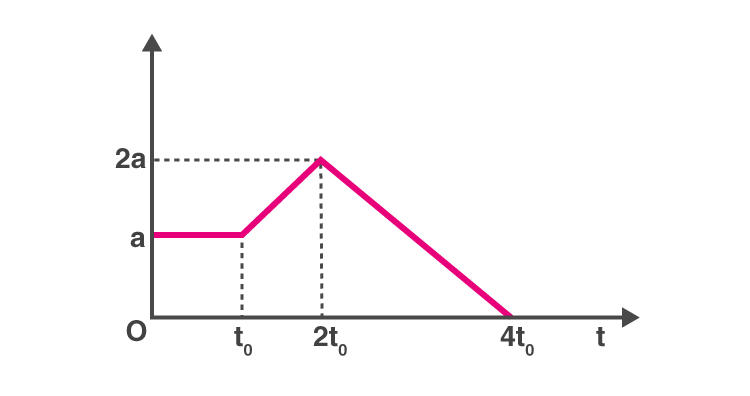## Physics MCQ on Kinematics for NEET, JEE, Medical and Engineering Exam 2021

MCQ on Kinematics: Previous trends reveal that NEET/JEE aspirants find Physics as the most challenging section amongst the other sections. But most of them fail to decipher the reason behind this. This particularly can be as the candidates taking up this examination are predominantly medical aspirants with their subject of interest being Biology. Also, sections like Physics are more on theories, laws, numerical as opposed to Biology which is more of fact-based, life sciences and comprising of substantial explanations.In this post we are providing you MCQ on Kinematics, which will be beneficial for you in upcoming NEET, AIIMS, JEE, WBJEE, Medical and Engineering Exams.

## MCQ on Kinematics

Q1. What will be the shape of the x→y graph, if the relation between time and displacement of the moving body is t = 2αx2 where α is constant.
a) Circle
b) Straight line
c) Parabola
d) Hyperbola

(d) Hyperbola

Q2. When can we say that the resultant of the two vectors is maximum ?
a) When the vectors are acting in the opposite direction
b) Both the vectors are acting in the same direction
c) When the vectors are at right angles
d) When the vectors are parallel to each other

(b) Both the vectors are acting in the same direction

Q3. Consider two cars M and N. These cars start from the same point and travel in a straight line such that their positions are xM(t) = at + bt2 and xN(t) = ft – t2. Determine the time at which both the cars are having the same velocity.
a) a−f/1+b
b) f−a/2(1+b)
c) a+f/2(b−1)
d) a+f/2(1+b)

(b) f−a/2(1+b)

Q4. What does the area under acceleration-time graph represent for any given time interval ?
a) Final velocity
b) Distance travelled
c) Change in the velocity in that time interval
d) Displacement of the particle

(c) Change in the velocity in that time interval

Q5. A particle with radius R is moving in a circular path with constant speed. The time period of the particle is T. Calculate the time for the following after t=T6. What is the average velocity of the particle.
a) 3R/T
b) 4R/T
c) 6R/T
d) 2R/T

(c) 6R/T

Q6. Below is the graph of a body whose initial velocity is v0. The body travels through a straight line with acceleration as shown in the graph. Determine the maximum velocity of the body.

a) v0-4at0b) v0+4at0
c) v0+(at0)
d) v0+9/2(at0)

(d) v0+9/2(at0)

Q7. Below is the distance-time curve traced by the particle. What is the maximum instantaneous velocity of the particle around the point.a) D
b) B
c) A
d) C

(c) C

Q8. Which of the following statement is true if a body moves in a semicircular track whose radius is R.
a) 2R is the displacement of the body
b)  πR is the distance travelled by the body
c) Both (a) and (b) are correct
d) None of the above.

(c) Both (a) and (b) are correct